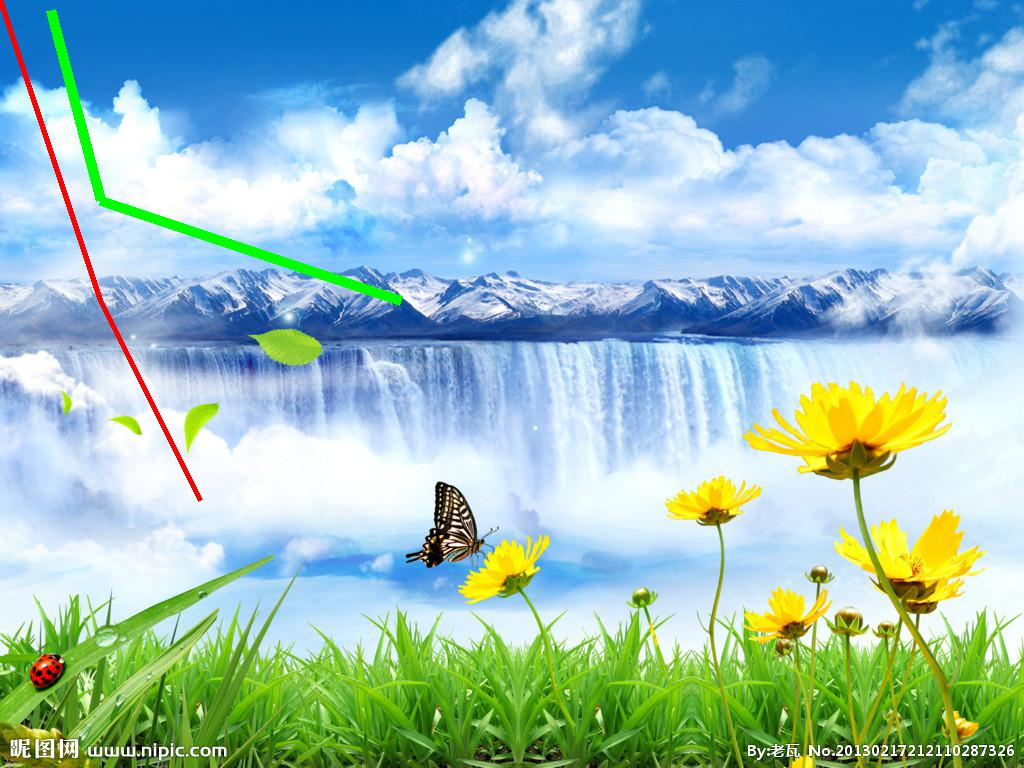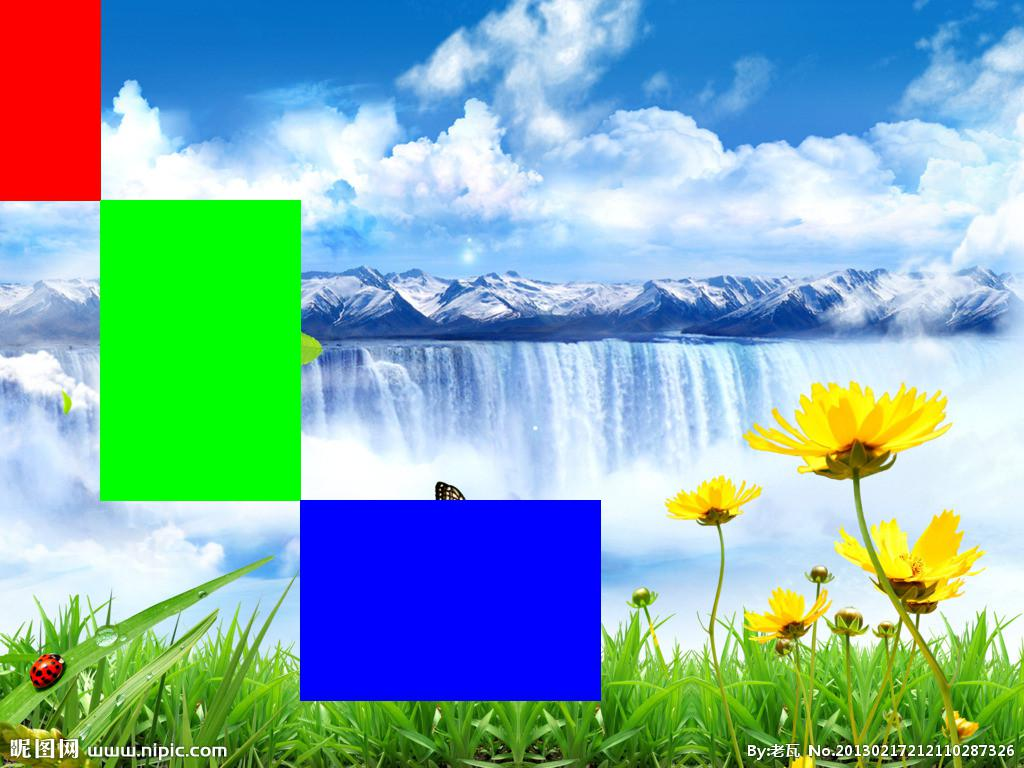# Python图像处理库PIL的ImageDraw模块介绍

ImageDraw模块提供了图像对象的简单2D绘制。用户可以使用这个模块创建新的图像，注释或润饰已存在图像，为web应用实时产生各种图形。

PIL中一个更高级绘图库见The aggdraw Module

1、  Coordinates

2、  Colours

3、  Colours Names

PIL 1.1.4及其以后的版本，用户绘制“RGB”图像时，可以使用字符串常量。PIL支持如下字符串格式：

A、 十六进制颜色说明符，定义为“#rgb”或者“#rrggbb”。例如，“#ff0000”表示纯红色。

B、 RGB函数，定义为“rgb(red, green, blue)”，变量redgreenblue的取值为之间的整数。另外，颜色值也可以为[0%100%]之间的三个百分比。例如，“rgb(255, 0, 0)”和“rgb(100%, 0%, 0%)”都表示纯红色。

C、 HSLHue-Saturation-Lightness）函数，定义为“hsl(hue,saturation%, lightness%)”，变量hue一个角度表示颜色（red=0 green=120 blue=240），变量saturation[0%100%]之间的一个值（gray=0%full color=100%），变量lightness[0%100%]之间的一个值（black=0% normal=50% white=100%）。例如，“hsl(0,100%, 50%)”为纯红色。

D、 通用HTML颜色名称，ImageDraw模块提供了140个标准颜色名称，Xwindow系统和大多数web浏览器都支持这些颜色。颜色名称对大小写不敏感。例如，“red”和“Red”都表示纯红色。

4、  Fonts

PIL可以使用bitmap字体或者OpenType/TrueType字体。

Bitmap字体被存储在PIL自己的格式中，它一般包括两个文件，一个叫.pil，它包含字体的矩阵，另一个通常叫做.pbm，它包含栅格数据。

ImageFont模块中，使用函数truetype()加载一个OpenType/TrueType字体。注意：这个函数依赖于第三方库，而且并不是在所有的PIL版本中都有效。

IronPIL）加载内置的字体，使用ImageFont模块的Font()结构函数即可。

1、  Draw

IronPIL）用户可以使用ImageWin模块的HWND或者HDC对象来代替图像。这个允许用户直接在屏幕上绘图。

>>> im01 =Image.open("D:\\Code\\Python\\test\\img\\test01.jpg")

>>> draw =ImageDraw.Draw(im01)

>>> draw.line((0,0) +im01.size, fill=128)

>>> draw.line((0,im01.size, im.size, 0), fill = 128)

>>> im01.show()

>>> del draw1、  Arc

>>> from PIL import Image,ImageDraw

>>> draw =ImageDraw.Draw(im01)

>>>draw.arc((300,300,500,500), 0, -90, fill = (0,255,0))

>>> im01.show()

>>> del draw2、  Bitmap

>>> from PIL import Image,ImageDraw

>>> im01 =Image.open("D:\\Code\\Python\\test\\img\\test01.jpg")

>>> im02 =Image.open("D:\\Code\\Python\\test\\img\\test02.jpg")

>>> im =im02.resize(300,200)>>> im.size

(300, 200)

>>> r,g,b =im.split()

>>> draw =ImageDraw.Draw(im01)

>>>draw.bitmap((0,0), r, fill = (255,0,0))

>>>draw.bitmap((300,200), g, fill = (0,255,0))

>>>draw.bitmap((600,400), b, fill = (0,0,255))

>>> im01.show()3、  Chord

>>> im01 =Image.open("D:\\Code\\Python\\test\\img\\test01.jpg")

>>> draw =ImageDraw.Draw(im01)

>>> draw.chord((0,0,200,200),0, 90, fill = (255,0,0))

>>> draw.chord((300,300,500,500), 0, -90, fill = (0,255,0))

>>> draw.chord((200,200,300,300), -90, 0, fill = (0,0,255))

>>> im01.show()4、  Ellipse

>>> im01 =Image.open("D:\\Code\\Python\\test\\img\\test01.jpg")

>>> draw =ImageDraw.Draw(im01)

>>> draw.ellipse((0,0, 200, 200), fill = (255, 0, 0))

>>> draw.ellipse((200,200,400,300),fill = (0, 255, 0))

>>>draw.ellipse((400,400,800,600), fill = (0, 0, 255))

>>> im01.show()5、  Line

New in 1.1.5）变量optionswidth给定线的宽度。注意线连接不是很好，所以多段线段连接不好看。

>>>from PIL import Image, ImageDraw

>>>im01 = Image.open("D:\\Code\\Python\\test\\img\\test01.jpg")

>>>draw = ImageDraw.Draw(im01)

>>>draw.line([(0,0),(100,300),(200,500)], fill = (255,0,0), width = 5)

>>>draw.line([50,10,100,200,400,300], fill = (0,255,0), width = 10)

>>>im01.show()6、  Pieslice

>>>from PIL import Image, ImageDraw

>>>im01 = Image.open("D:\\Code\\Python\\test\\img\\test01.jpg")

>>>draw = ImageDraw.Draw(im01)

>>>draw.pieslice((0,0,100,200), 0, 90, fill = (255,0,0))

>>>draw.pieslice((100,200,300,400), 0, -90, fill = (0,255,0))

>>> im01.show()7、  Point

>>>from PIL import Image, ImageDraw

>>>im01 = Image.open("D:\\Code\\Python\\test\\img\\test01.jpg")

>>>draw = ImageDraw.Draw(im01)

>>> draw.point([(0,0),(100,150), (110, 50)], fill = (255, 0, 0))

>>> draw.point([0,10,100,110, 210, 150], fill = (0, 255, 0))

>>>im01.show()8、  Polygon

>>>from PIL import Image, ImageDraw

>>>im01 = Image.open("D:\\Code\\Python\\test\\img\\test01.jpg")

>>>draw = ImageDraw.Draw(im01)

>>> draw.polygon([(0,0),(100,150), (210, 350)], fill = (255, 0, 0))

>>> draw.polygon([300,300,100,400, 400, 400], fill = (0, 255, 0))

>>>im01.show()9、  Rectangle

>>>from PIL import Image, ImageDraw

>>>im01 = Image.open("D:\\Code\\Python\\test\\img\\test01.jpg")

>>>draw = ImageDraw.Draw(im01)

>>>draw.rectangle((0,0,100,200), fill = (255,0,0))

>>> draw.rectangle([100,200,300,500],fill = (0,255,0))

>>>draw.rectangle([(300,500),(600,700)], fill = (0,0,255))

>>>im01.show()10、             Text

>>>from PIL import Image, ImageDraw

>>>im01 = Image.open("D:\\Code\\Python\\test\\img\\test01.jpg")

>>>draw = ImageDraw.Draw(im01)

>>> draw.text((0,0),"Hello", fill = (255,0,0))

>>>im01.show()

11、             Textsize

>>>from PIL import Image, ImageDraw

>>>im01 = Image.open("D:\\Code\\Python\\test\\img\\test01.jpg")

>>>draw = ImageDraw.Draw(im01)

>>>draw.textsize("Hello")

(30, 11)

>>>draw.textsize("Hello, the world")

(96, 11)

>>>im01.show()

Option变量有三个属性，分别为outlinefillfontOutlinefill都可为整数或者元组；fontImageFont类的实例。

Draw包括的一个构造函数和一些方法提供向后兼容。为了使这些函数正常工作，用户应该使用options，或者使用这些方法。但不能混合旧的和新的调用习惯。

IronPILIronPIL不支持这些有兼容性的方法。

1、  ImageDraw

2、  Setink

3、   Setfill

4、  Setfill©️2019 CSDN 皮肤主题: 大白 设计师: CSDN官方博客